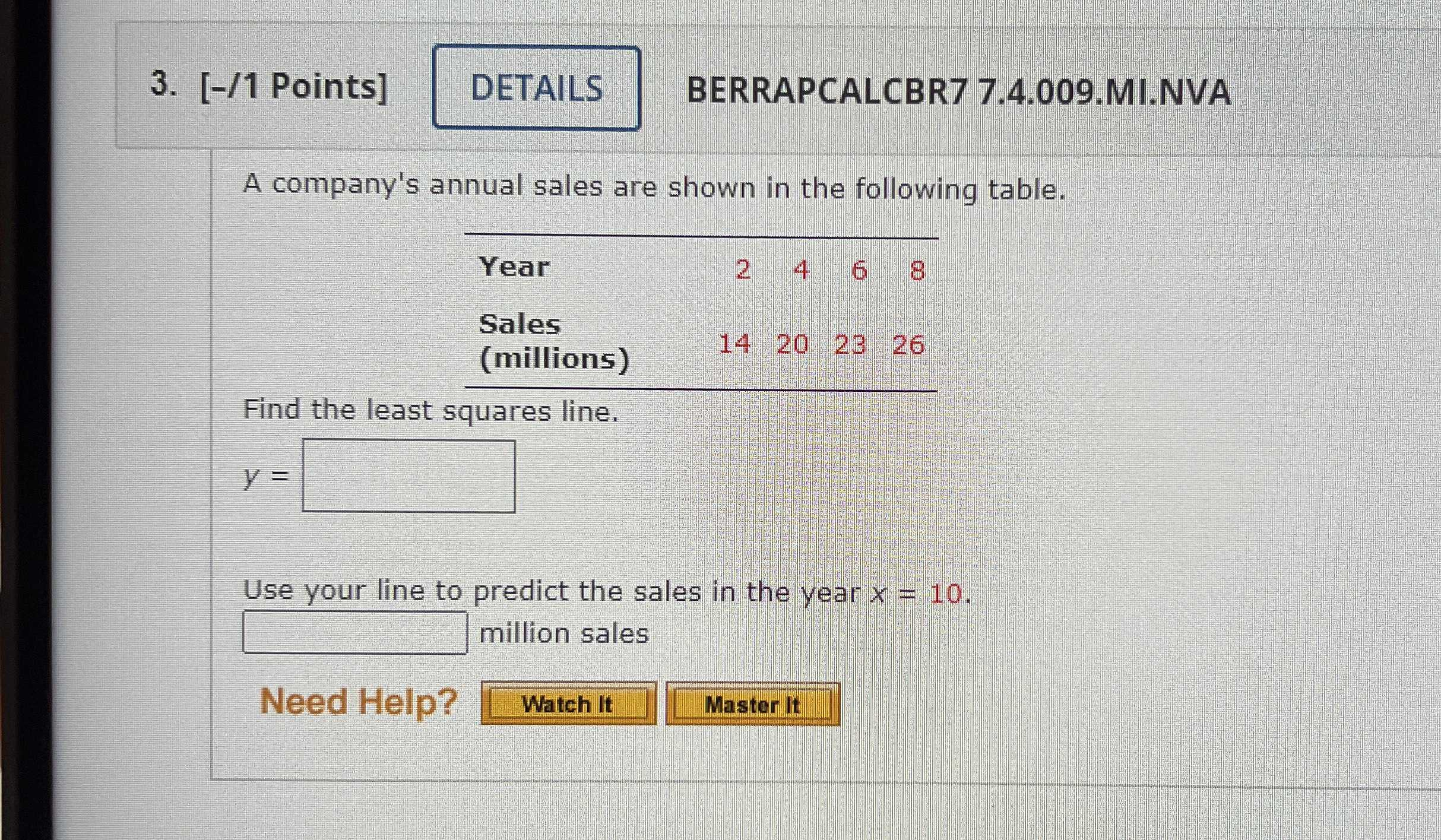### ¿Todavía tienes preguntas de matemáticas?

Pregunte a nuestros tutores expertos
Algebra
PreguntaA company's annual sales are shown in the following table. Find the least squares line. $$y = \square$$. Use your line to predict the sales in the year $$x = 10$$ . $$\square$$ million sales

$$y= 1.95x+ 11$$